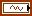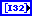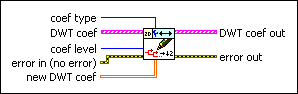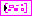# WA Set Coefficients of Discrete Wavelet Transform VI

LabVIEW 2014 Advanced Signal Processing Toolkit Help

Edition Date: June 2014

Part Number: 372656C-01

»View Product InfoDownload Help (Windows Only)

Owning Palette: Discrete Wavelet VIs

Sets approximation or detail discrete wavelet transform (DWT) coefficients at a specific level. Use the WA Discrete Wavelet Transform VI to compute the DWT. Use the WA Get Coefficients of Discrete Wavelet Transform VI to obtain the DWT coefficients.

Examples

Use the pull-down menu to select an instance of this VI.

 Select an instance WA Set Coefficients of 1D Discrete Wavelet Transform (Waveform)WA Set Coefficients of 1D Discrete Wavelet Transform (Array)WA Set Coefficients of 2D Discrete Wavelet Transform

## WA Set Coefficients of 1D Discrete Wavelet Transform (Waveform)coef type specifies whether this VI sets the approximation coefficients or detail coefficients. The default is Approximation coefficients.DWT coef specifies the approximation coefficients and the detail coefficients from the multi-level discrete wavelet transform (DWT). You must organize the coefficients into a waveform starting with the approximation coefficients at the largest level followed by the detail coefficients at all levels in descending order. If you use the WA Get Coefficients of Discrete Wavelet Transform VI to obtain the DWT coefficients, wire the DWT coef out output of the WA Get Coefficients of Discrete Wavelet Transform VI to the DWT coef input of this VI.length specifies, in a 1D array, the number of approximation and detail coefficients at each level. At a decomposition level of N, length is equal to N+2. The first element of length always is equal to the number of approximation coefficients. The last element of length indicates the total number of raw data samples. You must arrange the length of the detail coefficients in descending order. If you use the WA Get Coefficients of Discrete Wavelet Transform VI to obtain the DWT coefficients, wire the length out output of the WA Get Coefficients of Discrete Wavelet Transform VI to the length input of this VI.coef level specifies the coefficient level this VI sets. The coef level must be between 1 and the largest level of the detail coefficients. The levels in the WA Discrete Wavelet Transform VI specifies the largest level of the coefficients. The default is 1, which means this VI sets the coefficients at level 1. If coef type is Approximation coefficients, this VI ignores coef level. If coef type is Detail coefficients, this VI sets the coefficients at the level you specify in coef level.error in describes error conditions that occur before this node runs. This input provides standard error in functionality.new DWT coef specifies the discrete wavelet transform (DWT) coefficients to set.DWT coef out returns the discrete wavelet transform (DWT) coefficients set to new DWT coef at the coef type and coef level.length out returns length unchanged.error out contains error information. This output provides standard error out functionality.

## WA Set Coefficients of 1D Discrete Wavelet Transform (Array)coef type specifies whether this VI sets the approximation coefficients or detail coefficients. The default is Approximation coefficients.DWT coef specifies the approximation coefficients and the detail coefficients from the multi-level discrete wavelet decomposition (DWT). You must organize the coefficients into a 1D real array starting with the approximation coefficients at the largest level followed by the detail coefficients at all levels in descending order. If you use the WA Get Coefficients of Discrete Wavelet Transform VI to obtain the DWT coefficients, wire the DWT coef out output of the WA Get Coefficients of Discrete Wavelet Transform VI to the DWT coef input of this VI.length specifies, in a 1D array, the number of approximation and detail coefficients at each level. At a decomposition level of N, length is equal to N+2. The first element of length always is equal to the number of approximation coefficients. The last element of length indicates the total number of raw data samples. You must arrange the length of the detail coefficients in descending order. If you use the WA Get Coefficients of Discrete Wavelet Transform VI to obtain the DWT coefficients, wire the length out output of the WA Get Coefficients of Discrete Wavelet Transform VI to the length input of this VI.coef level specifies the coefficient level this VI sets. The coef level must be between 1 and the largest level of the detail coefficients. The levels in the WA Discrete Wavelet Transform VI specifies the largest level of the coefficients. The default is 1, which means this VI sets the coefficients at level 1. If coef type is Approximation coefficients, this VI ignores coef level. If coef type is Detail coefficients, this VI sets the coefficients at the level you specify in coef level.error in describes error conditions that occur before this node runs. This input provides standard error in functionality.new DWT coef specifies the discrete wavelet transform (DWT) coefficients to set.DWT coef out returns the discrete wavelet transform (DWT) coefficients set to new DWT coef at the coef type and coef level.length out returns length unchanged.error out contains error information. This output provides standard error out functionality.

## WA Set Coefficients of 2D Discrete Wavelet Transformcoef type specifies the coefficient type this VI sets.

 0 low_low (default)—Specifies the approximation coefficients from the lowpass analysis filtering on each row and each column. The low_low coefficients are a low-resolution approximation of the original 2D signal. 1 low_high—Specifies the detail coefficients from the lowpass analysis filtering on each row and the highpass analysis filtering on each column. The high-frequency signal along the column direction influences the low_high coefficients. 2 high_low—Specifies the detail coefficients from the highpass analysis filtering on each row and the lowpass analysis filtering on each column. The high-frequency signal along the row direction influences the high_low coefficients. 3 high_high—Specifies the detail coefficients from the highpass analysis filtering on each row and each column. The high-frequency signal along the diagonal direction influences the high_high coefficients.DWT coef specifies the approximation coefficients and the detail coefficients from the multi-level discrete wavelet transform. Each element of the array contains the 2D DWT results of one level. The ith element stores the approximation coefficients and the detail coefficients at level i+1. If you use the WA Get Coefficients of Discrete Wavelet Transform VI to obtain the DWT coefficients, wire the DWT coef out output of the WA Get Coefficients of Discrete Wavelet Transform VI to the DWT coef input of this VI.low_low specifies the approximation coefficients from the lowpass analysis filtering on each row and each column. The low_low coefficients are a low-resolution approximation of the original 2D signal.low_high specifies the detail coefficients from the lowpass analysis filtering on each row and the highpass analysis filtering on each column. The high-frequency signal along the column direction influences the low_high coefficients.high_low specifies the detail coefficients from the highpass analysis filtering on each row and the lowpass analysis filtering on each column. The high-frequency signal along the row direction influences the high_low coefficients.high_high specifies the detail coefficients from the highpass analysis filtering on each row and each column. The high-frequency signal along the diagonal direction influences the high_high coefficients.coef level specifies the coefficient level this VI sets. The coef level must be between 1 and the largest level of the coefficients. The levels in the WA Discrete Wavelet Transform VI specifies the largest level of the coefficients. The default is 1, which means this VI sets the coefficients at level 1.error in describes error conditions that occur before this node runs. This input provides standard error in functionality.new DWT coef specifies the discrete wavelet transform (DWT) coefficients to set.DWT coef out returns the discrete wavelet transform (DWT) coefficients set to new DWT coef at the coef type and coef level.low_low out returns the approximation coefficients from the lowpass analysis filtering on each row and each column. The low_low out coefficients are a low-resolution approximation of the original 2D signal.low_high out returns the detail coefficients from the lowpass analysis filtering on each row and the highpass analysis filtering on each column. The high-frequency signal along the column direction influences the low_high out coefficients.high_low out returns the detail coefficients from the highpass analysis filtering on each row and the lowpass analysis filtering on each column. The high-frequency signal along the row direction influences the high_low out coefficients.high_high out returns the detail coefficients from the highpass analysis filtering on each row and each column. The high-frequency signal along the diagonal direction influences the high_high out coefficients.error out contains error information. This output provides standard error out functionality.

## Examples

Refer to the following VIs for examples of using the WA Set Coefficients of Discrete Wavelet Transform VI:

• Get and Set Approximation Coefficients VI: labview\examples\Wavelet Analysis\WAGettingStarted
• Get and Set Single Level Detail Coefficients VI: labview\examples\Wavelet Analysis\WAGettingStarted